# CBSE Class 10 Physics Electricity Assignment Set C

Read and download free pdf of CBSE Class 10 Physics Electricity Assignment Set C. Get printable school Assignments for Class 10 Physics. Class 10 students should practise questions and answers given here for Chapter 12 Electricity Physics in Class 10 which will help them to strengthen their understanding of all important topics. Students should also download free pdf of Printable Worksheets for Class 10 Physics prepared as per the latest books and syllabus issued by NCERT, CBSE, KVS and do problems daily to score better marks in tests and examinations

## Assignment for Class 10 Physics Chapter 12 Electricity

Class 10 Physics students should refer to the following printable assignment in Pdf for Chapter 12 Electricity in Class 10. This test paper with questions and answers for Class 10 Physics will be very useful for exams and help you to score good marks

### Chapter 12 Electricity Class 10 Physics Assignment

Question : Four different measuring instruments are shown below. Out of these, the instrument that can be used for measuring current is/are the instruments labelled as(a) II and IV with IV more reliable of the two
(b) II and III with II more reliable of the two
(c) I and III with III more reliable of the two
(d) I and IV with IV more reliable of the two

Explanation: Option with 4 and 2 is wrong as they are with voltmeters. Option I is nearest. The best option may be I and III with III more accuracy of the two since L.C. is less.

Question : Three students X, Y and Z, while performing the experiment to study the dependence of current on the potential difference across a resistor, connect the ammeter (A), the battery (B), the key (K) and the resistor (R), in series, in the following three different orders.
X → B, K, R, A, B
Y → B, A, K,R,B
Z → B, R, K, A, B
Who has connected them in the correct order ?
(a) Z
(b) X
(c) X, Y and Z
(d) Y

Explanation: The current in the series does not depend upon order in which these instruments are connected. Where they are connected does not matter.
They should be in series for the flow to be observed.

Question : Which of the following fuse should be used for an electric iron of 1 kW when operated at 220 V?
(a) 1 A
(b) 3 A
(c) 7 A
(d) 5 A

Question. A cylindrical conductor of length l and uniform area of cross section A has resistance R.
Another conductor of length 2l and resistance R of the same material has area of cross-section
(a) A/2
(b) 3A/2
(c) 2A
(d) 3A

Question. Specific resistance is numerically equal to the resistance offered by
(a) 1 cm length of a conductor
(b) a conductor of unit cross-section
(c) 1 cm length of conductor of 1 cm2 of crosssection
(d) 1 cm3 of a conductor

Question. 1 volt = ..............
(a) 1 joule / coulomb
(b) 1 coulomb/joule
(c) 1 joule/coulomb2
(d) 1 joule coulomb

Question. In the given circuit voltmeter shows a reading of 4 V, then the power developed across R resistance will be
(a) 15 mW
(b) 14 mW
(c) 12 mW
(d) 10 mW

Question. If current through a resistance is increased by 100%, simultaneously reducing resistance value to 25%, the new power dissipated will be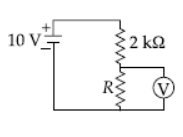(a) same
(b) increased by 100%
(c) decreased by 400%
(d) increased by 400%.

Question. The amount of heat produced in a conductor is
(a) directly proportional to the current flowing through it
(b) inversely proportional to the current flowing through it
(c) directly proportional to the square of the current flowing through it
(d) inversely proportional to the square of current flowing through it.

Question. A multimeter is used to measure
(a) current only
(b) resistance only
(c) voltage only
(d) current, resistance and voltage.

Question. The proper representation of series combination of cells obtaining maximum potential isQuestion. The V-I graph of resistor is shown in figure. If the resistance is determined at points A, B and C, then it is found that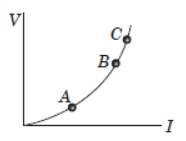(a) resistances at A, B and C a are equal
(b) resistance at C is more than that at B
(c) resistance at B is lower than that at A.
(d) resistance at C is lower than that at A.

Question. Suppose five resistances, each of 10 ohm, are provided to you. You are free to get the desired value by combining them. The desired value will lie in between
(a) 2 ohm to 50 ohm
(b) 20 ohm to 40 ohm
(c) 12 ohm to 50 ohm
(d) 10 ohm to 60 ohm

Question. In the following circuits, heat produced in the resistor or combination of resistors connected to a 12 V battery will be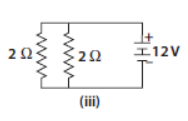(a) same in all the cases
(b) minimum in case (i)
(c) maximum in case (ii)
(d) maximum in case (iii)

Question. Masses of three wires of copper are in the ratio 1 : 3 : 5 and their lengths are in the ratio of 5 : 3 : 1. The ratio of their electrical resistances are
(a) 1 : 3 : 5
(b) 5 : 3 : 1
(c) 1 : 15 : 125
(d) 125 : 15 : 1

Question. If a wire of resistance R is melted and recast to half its length, the new resistance of the wire will be
(a) R/4
(b) R/2
(c) R
(d) 2R

Question. The given figure shows the I-V curve (i) for a nichrome wire of given length and cross-section. Which of the following will yield the curve (ii)?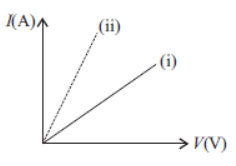(a) Increase the length of nichrome wire.
(b) Decrease the thickness of nichrome wire.
(c) Replace the nichrome wire with a similar copper wire.
(d) Replace the nichrome wire with a similar silicon wire.

Question. In a metallic conductor, electric current thought to be due to the movement of
(a) ions
(b) amperes
(c) electrons
(d) protons

Question. In order to distribute a high potential, we connect a number of resistors
(a) in series
(b) in parallel
(c) some in series and some in parallel
(d) It is not possible to distribute potential.

Question : Name the physical quantity whose unit is J/C.
Answer : Electric potential. It is represented by V.

Question : Why closed path is required for the flow of current?
Answer : A closed path is required for the flow of current so that charges can move in a particular direction in a given circuit. But if path is not closed means circuit is open, then there is air between the gap, and we know that air is an insulator for flow of charges, so the current stop flowing in the circuit.

Question : What is resistance of dry air?
Answer :  The resistance of dry air is infinity.

Question :`Draw a circuit diagram using a battery of two cells, two resistors of 3 each connected in series, a plug key and a rheostat.
Answer :   When we connect rheostat, key, battery of two cells and two resistors of three ohm each then required circuit diagram will be: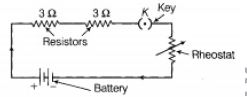Question : An electric motor takes 5A from a 220V line. Determine the power of the motor and the energy consumed in 2 h.
Answer :  Power = P = VI = 220 × 5 = 1,100 Ω
Energy consumed in 2 h (2 × 60 × 60s) E = Pt
E = (1,100) (2 × 60 × 60) = 7,920,000 J
E = 7.92 × 106 J.

Question : An electric lamp of 100 ohms, a toaster of resistance 50 ohms and a water filter of resistance 500 ohms are connected in parallel to a 220V source. what is the resistance of the electric iron connected to the same source that takes as much current as all the three appliances and what is the current through it ?
Answer : Combined resistnace of 100Ω, 50Ω and 500Ω in parallel i.e. Rp is given byQuestion : What is the current through each of the resistances in the following circuit ?Resistors 1Ω and 2 Ω are in parallel.Question : Two metallic wires A and B are connected in series. Wire A has length l and radius r, while wire B has length 2l and radius 2r. Find the ratio of total resistance of series combination and the resistance of wire A, if both the wires are of the same material?Question : Redraw the circuit putting an ammeter to measure the current through the resistors and voltmeter to measure the potential difference across 12 Ω resistor. what would be the reading in the ammeter and the voltmeter?
Modified circuit is as shown. Since 5Ω, 8 Ω and 12 Ω are in series, therefore the total resistance in series.
Rs = R1 + R2 + R3 = 5 + 8 + 12 = 25Ω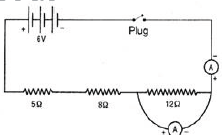Current through circuit I = V/R = 6/25 = 0.24A
= I. R.= 0.24 x 12
= 2.88Ω

Question : Three resistors are connected as shown in Fig. Through a resistor of 5 ohms; a current of 1A is flowing.
i. what is the current through the other two resistors?
ii. what is the potential difference (P.D.) across AB and AC?
iii. what is the total resistance?Answer :  Let us first find the total resistance. Now resistance of 10 Ω and 15 Ω are in parallel.
If Rp is the effective resistance between B and C, then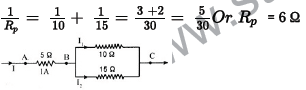Again AB and BC are in series.
Therefore, the total resistance = Resistance between A and B plus resistance between B and C.
i.e. Total resistance = 5 Ω + 6 Ω = 11 Ω
Potential difference between A and B = IR = 1 x 5 = 5 V
Potential difference between B and C = 1 x 6 = 6 V
Potential difference between A and C = 1 x 11 = 11 V
Current through A and B = 1 A
This current divides into two parts, one part I1 passing through 10 Ω and other part I2
passing through 15 Ω each producing a P.D. of 6V (between B and C)
I1 (10) = 6 or I1 = 6/10 = 0.6 A and I2(15) = 6 or I2 = 6/15 = 0.4 A

Question : How do we express electric current?

Question : What is an electric circuit?

Question : What does a switch do?

Question : Conventionally, in an electric circuit the direction of electric current is taken as opposite to the direction of the flow of electrons, which are negative charges. Why?

Question : What is the SI unit of electric charge? How many electrons make one coulomb of charge?

Question : Define the SI unit of current.

Question : Which instrument is used to measure the current flowing in a circuit?How is it connected in the circuit and why?

Question : In which smaller units can we measure the small amount of current flowing in the circuit?

Question : How are the related to the SI unit of current?

Question : What makes the electric charge to flow?

Please click the below link to access CBSE Class 10 Physics Electricity Assignment Set C

 CBSE Class 10 Science Chemical Reactions and Equations Assignment
 CBSE Class 10 Science Acids Bases and Salts Assignment Set A CBSE Class 10 Science Acids Bases and Salts Assignment Set B CBSE Class 10 Science Acids Bases and Salts Assignment Set C
 CBSE Class 10 Science Metals and Non Metals Assignment Set A CBSE Class 10 Science Metals and Non Metals Assignment Set B
 CBSE Class 10 Science Carbon and its compounds Assignment Set A CBSE Class 10 Science Carbon and its compounds Assignment Set B CBSE Class 10 Science Carbon and its compounds Assignment Set C CBSE Class 10 Science Carbon and its compounds Assignment Set D CBSE Class 10 Science Carbon and its compounds Assignment Set E
 CBSE Class 10 Science Periodic Classification Of Elements Assignment Set A CBSE Class 10 Science Periodic Classification Of Elements Assignment Set B CBSE Class 10 Science Periodic Classification Of Elements Assignment Set C
 CBSE Class 10 Science Biology Life Process Respiration Assignment CBSE Class 10 Science Biology Nutrition Assignment CBSE Class 10 Science Biology Respiration Assignment CBSE Class 10 Science Biology Transport Enrichment Assignment CBSE Class 10 Science Biology Transportation Assignment CBSE Class 10 Science Life Processes Assignment Set A CBSE Class 10 Science Life Processes Assignment Set B CBSE Class 10 Science Life Processes Assignment Set C
 CBSE Class 10 Science Control and Coordination Assignment Set A CBSE Class 10 Science Control and Coordination Assignment Set B CBSE Class 10 Science Control and Coordination Assignment Set C CBSE Class 10 Science Control and Coordination Assignment Set D
 CBSE Class 10 Science How do Organisms Reproduce Assignment Set A CBSE Class 10 Science How do Organisms Reproduce Assignment Set B
 CBSE Class 10 Science Heredity and Evolution Assignment Set A CBSE Class 10 Science Heredity and Evolution Assignment Set B CBSE Class 10 Science Heredity and Evolution Assignment Set C
 CBSE Class 10 Science Light Reflection and Refraction Assignment Set A CBSE Class 10 Science Light Reflection and Refraction Assignment Set B CBSE Class 10 Science Light Reflection and Refraction Assignment Set C CBSE Class 10 Science Light Reflection and Refraction Assignment Set D CBSE Class 10 Science Light Reflection and Refraction Assignment Set E CBSE Class 10 Science Light Reflection and Refraction Assignment Set F
 CBSE Class 10 Science Human Eye and Colorful World Assignment Set A CBSE Class 10 Science Human Eye and Colorful World Assignment Set B CBSE Class 10 Science Human Eye and Colorful World Assignment Set C
 CBSE Class 10 Physics Electricity Assignment Set A CBSE Class 10 Physics Electricity Assignment Set B CBSE Class 10 Physics Electricity Assignment Set C CBSE Class 10 Science Electricity Assignment Set A CBSE Class 10 Science Electricity Assignment Set B CBSE Class 10 Science Electricity Assignment Set C
 CBSE Class 10 Science Magnetic Effects of Electric Current Assignment Set A CBSE Class 10 Science Magnetic Effects of Electric Current Assignment Set B CBSE Class 10 Science Magnetic Effects of Electric Current Assignment Set C CBSE Class 10 Science Magnetic Effects of Electric Current Assignment Set D
 CBSE Class 10 Science Sources of Energy Assignment Set A CBSE Class 10 Science Sources of Energy Assignment Set B CBSE Class 10 Science Sources of Energy Assignment Set C CBSE Class 10 Science Sources of Energy Assignment Set D
 CBSE Class 10 Science Our Environment Assignment Set A CBSE Class 10 Science Our Environment Assignment Set B CBSE Class 10 Science Our Environment Assignment Set C
 CBSE Class 10 Science Sustainable Management of Natural Resources Assignment Set A CBSE Class 10 Science Sustainable Management of Natural Resources Assignment Set B CBSE Class 10 Science Sustainable Management of Natural Resources Assignment Set C
 CBSE Class 10 Physics Revision Assignment Set A CBSE Class 10 Physics Revision Assignment Set B CBSE Class 10 Physics Revision Assignment Set C CBSE Class 10 Physics Revision Assignment Set D CBSE Class 10 Physics Revision Assignment Set E CBSE Class 10 Physics Revision Assignment Set F CBSE Class 10 Physics Revision Assignment Set G CBSE Class 10 Physics Revision Assignment Set H
 CBSE Class 10 Science Assignment CBSE Class 10 Science Assignments Collection CBSE Class 10 Science Energy Crossword Puzzle Assignment
 CBSE Class 10 Physics Question Bank CBSE Class 10 Physics Tungsten Assignment
 CBSE Class 10 Science Chemistry Cleaning Capacity of Soap Assignment CBSE Class 10 Science Chemistry Determination of pH Assignment CBSE Class 10 Science Chemistry Different Types of Chemical Reactions Assignment CBSE Class 10 Science Chemistry Displacement Reaction and Reactivity Series Assignment CBSE Class 10 Science Chemistry Properties of Acetic Acid Assignment CBSE Class 10 Science Chemistry Saponification Preparation of Soap Assignment CBSE Class 10 Science Chemistry Study of properties of HCI Acid and NaOH Assignment

## More Study Material

Tags

### CBSE Class 10 Physics Chapter 12 Electricity Assignment

We hope you liked the above assignment for Chapter 12 Electricity which has been designed as per the latest syllabus for Class 10 Physics released by CBSE. Students of Class 10 should download and practice the above Assignments for Class 10 Physics regularly. We have provided all types of questions like MCQs, short answer questions, objective questions and long answer questions in the Class 10 Physics practice sheet in Pdf. All questions have been designed for Physics by looking into the pattern of problems asked in previous year examinations.

#### Assignment for Physics CBSE Class 10 Chapter 12 Electricity

Our team of expert teachers have referred to NCERT book for Class 10 Physics to design the Physics Class 10 Assignments. If you practice at least one test paper daily, you will get higher marks in Class 10 exams this year. Daily practice of Physics course notes and related study material will help you to clear all your doubts and have stronger understanding of all concepts. You can download all Revision notes for Class 10 Physics also from www.studiestoday.com absolutely free of cost.

#### Chapter 12 Electricity Assignment Physics CBSE Class 10

All questions and their answers for the assignment given above for Class 10 Physics have been developed as per the latest curriculum and books issued for the current academic year. The students of Class 10 can rest assured that the best teachers have designed the questions of Physics so that you are able to revise the entire syllabus if you do the assignments. Lot of MCQ questions for Class 10 Physics have also been given in the worksheets and assignments for regular use. All study material for Class 10 Physics students have been given on studiestoday.

#### Chapter 12 Electricity Assignment CBSE Class 10 Physics

Regular assignment practice helps to get a more comprehensive understanding of Chapter 12 Electricity concepts. Assignments play a crucial role in understanding Chapter 12 Electricity in CBSE Class 10. Students can download all the assignments of the same chapter in Class 10 Physics in Pdf format. You can print them or read them online on your computer or mobile.

#### CBSE Physics Class 10 Chapter 12 Electricity Assignment

CBSE Class 10 Physics latest books have been used for coming up with the latest questions and solutions for the above assignment. If you have revised all concepts relating to Chapter 12 Electricity then you should attempt all questions given in the test sheets above. We have also provided lot of Worksheets for Class 10 Physics which you can use to further make your self stronger in Physics

Where can I download in PDF assignments for CBSE Class 10 Physics Chapter 12 Electricity

You can download free Pdf assignments for CBSE Class 10 Physics Chapter 12 Electricity from StudiesToday.com

The assignments for Chapter 12 Electricity Class 10 Physics for have been made based on which syllabus

The Chapter 12 Electricity Class 10 Physics Assignments have been designed based on latest CBSE syllabus for Class 10 Physics issued for the current academic year

Can I download and print these printable assignments for Physics Chapter 12 Electricity Class 10

Yes, These printable assignments for Chapter 12 Electricity Class 10 Physics are free to download and print

How many topics are covered in Chapter 12 Electricity Physics assignments for Class 10

All topics given in Chapter 12 Electricity Physics Class 10 Book for the current academic year have been covered in the given assignment

Is there any charge for this assignment for Chapter 12 Electricity Physics Class 10

No, all Printable Assignments for Chapter 12 Electricity Class 10 Physics have been given for free and can be downloaded in Pdf format

How can I download the printable test assignments for Chapter 12 Electricity Physics Class 10

Just click on the View or Download button below, then another window with the Pdf will be visible, just click on the Pdf icon to download the free assignments for Chapter 12 Electricity Class 10 Physics

Are these assignments available for all chapters in Class 10 Physics

Yes, apart from Physics you can download free assignments for all subjects in Class 10

Can I download solved assignments for Chapter 12 Electricity CBSE Class 10 Physics

Our team of expert teachers at studiestoday.com have provided all answers for the practice questions which have been given in Class 10 Physics Chapter 12 Electricity assignments